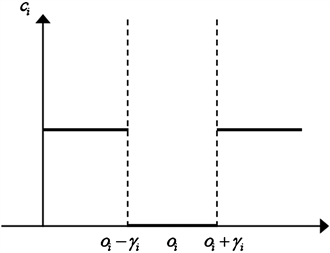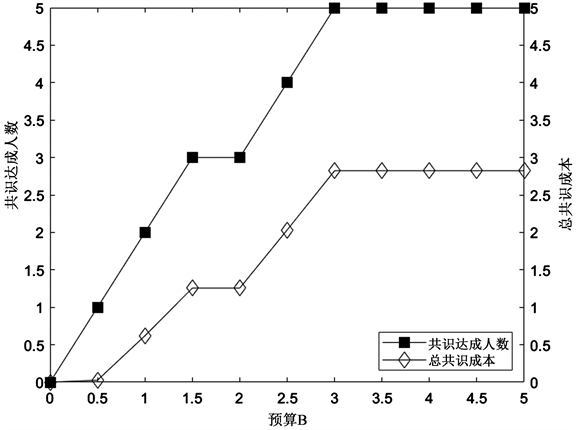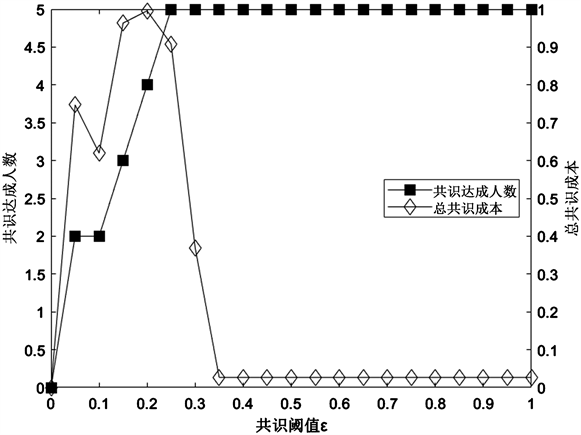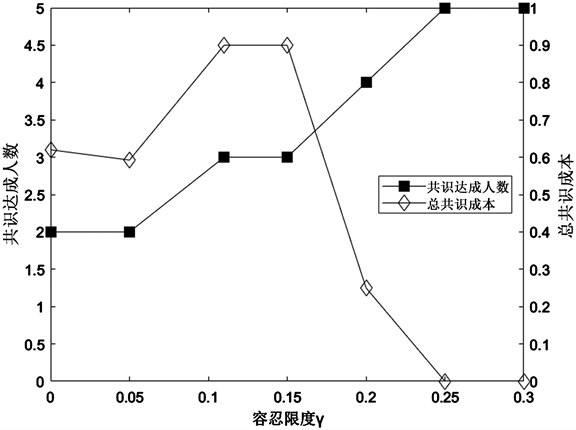#### 期刊菜单

Research on Maximum Experts Consensus Model Considering the Association among Decision-Makers and the Tolerance Behavior

Abstract: The aim of the maximum experts consensus is to maximize the number of decision-makers (DMs) who are reached the consensus under the limited budget. The weight of the DMs is often given by the moderator, which is subjective. In addition, existing research has ignored the impact of DMs’ behavior on consensus results. In this paper, we study the method of determining the weight of DMs through the evaluation of associated attributes and the maximum experts consensus problem considering the DMs’ tolerant behavior. First, consider the correlation between evaluation attributes and use the Choquet integral to determine the weight of DMs. Secondly, put emphasis on the moderator’s opinion and the DMs’ tolerant behavior; then build a maximum experts consensus model considering the DMs’ tolerance limit. Finally, the validity of the model is verified by an example. The results show that: 1) The DMs’ tolerant behavior is conducive to reaching the maximum experts consensus. 2) The weight determination method considering associated attributes can reduce the influence of subjective preference. 3) The moderator’s opinion can reasonably regulate the consensus opinions.

1. 引言

2. 相关理论基础

2.1. 模糊测度理论

1) ${g}_{\lambda }:P\left(A\right)\to \left[0,1\right]$${g}_{\lambda }\left(\varnothing \right)=0,{g}_{\lambda }\left(A\right)=0$ (有界性)

2) $\forall {S}_{1},{S}_{2}\in P\left(A\right)$，若 ${S}_{1}\subseteq {S}_{2}$，则 ${g}_{\lambda }\left({S}_{1}\right)\le {g}_{\lambda }\left({S}_{2}\right)$ (单调性)

3) ${g}_{\lambda }\left({S}_{1}\cup {S}_{2}\right)={g}_{\lambda }\left({S}_{1}\right)+{g}_{\lambda }\left({S}_{2}\right)+\lambda {g}_{\lambda }\left({S}_{1}\right){g}_{\lambda }\left({S}_{2}\right),\lambda >-1$

${g}_{\lambda }$ 称为定义在A上的 $\lambda$ 模糊测度。

$g\left(S\right)=\left\{\begin{array}{ll}\frac{1}{\lambda }\left[\underset{j\in S}{\prod }\left(1+\lambda g\left(j\right)\right)-1\right]\hfill & 若\text{ }\text{ }\lambda \ne 0,\text{\hspace{0.17em}}-1<\lambda <+\infty \hfill \\ \underset{j\in S}{\sum }g\left(j\right)\hfill & 若\text{ }\text{ }\lambda =0\hfill \end{array}$ (1)

$\lambda +1=\underset{j=1}{\overset{n}{\prod }}\left(1+\lambda g\left(j\right)\right)$ (2)

2.2. 模糊积分理论

$CI=\int f\text{d}u=\underset{i=1}{\overset{n}{\sum }}\left(f\left({n}_{\left(i\right)}\right)-f\left({n}_{\left(i-1\right)}\right)\right)\mu \left({N}_{\left(i\right)}\right)$ (3)

2.3. 最大专家数共识模型

$D=\left\{{d}_{1},{d}_{2},\cdots ,{d}_{m}\right\}$ 为m个决策者的集合， $O=\left\{{o}_{1},{o}_{2},\cdots ,{o}_{m}\right\}$ 为决策者初始意见的集合， $\stackrel{¯}{O}=\left\{{\stackrel{¯}{o}}_{1},{\stackrel{¯}{o}}_{2},\cdots ,{\stackrel{¯}{o}}_{m}\right\}$ 为决策者调整后意见的集合，其中 ${d}_{i}$ 表示第i个决策者， ${o}_{i}$ 表示第i个决策者的初始意见， ${\stackrel{¯}{o}}_{i}$ 表示第i个决策者的调整后意见。通过WA算子聚合可得群体共识意见，我们定义为 ${o}_{c}$。令 $\epsilon$ 表示软共识阈值，当 $|{\stackrel{¯}{o}}_{i}-{\stackrel{¯}{o}}_{c}|\le \epsilon$ 时，则认为 ${d}_{i}$ 满足共识要求。给定 ${d}_{i}$ 单位调整成本为 ${c}_{i}$，则总共识成本为 ${\sum }_{i=1}^{n}{c}_{i}|{o}_{i}-{\stackrel{¯}{o}}_{i}|$。给定共识预算B，Zhang等  提出了在有限预算下最大化共识达成人数的最大专家数共识模型：

$\begin{array}{l}\mathrm{max}\underset{i=1}{\overset{n}{\sum }}{x}_{i}\\ \text{s}\text{.t}\text{.}\left\{\begin{array}{ll}\underset{i=1}{\overset{n}{\sum }}{c}_{i}|{\stackrel{¯}{o}}_{i}-{o}_{i}|\le B\hfill & \left(4\text{-}1\right)\hfill \\ {\stackrel{¯}{o}}_{c}=F\left({\stackrel{¯}{o}}_{1},{\stackrel{¯}{o}}_{2},\cdots ,{\stackrel{¯}{o}}_{n}\right)\hfill & \left(4\text{-}2\right)\hfill \\ {x}_{i}=\left\{\begin{array}{cc}1& \text{if}\text{\hspace{0.17em}}|{\stackrel{¯}{o}}_{i}-{\stackrel{¯}{o}}_{c}|\le \epsilon \\ 0& \text{else}\end{array}\hfill & \left(4\text{-}3\right)\hfill \\ i=1,2,\cdots ,n\hfill & \left(4\text{-}4\right)\hfill \end{array}\end{array}$ (4)

3. 考虑属性关联的决策者权重分配

$E=\left[\begin{array}{cccc}{e}_{11}& {e}_{12}& \cdots & {e}_{1n}\\ {e}_{21}& {e}_{22}& \cdots & {e}_{2n}\\ ⋮& ⋮& & ⋮\\ {e}_{m1}& {e}_{m2}& \cdots & {e}_{mn}\end{array}\right]$ (5)

${p}_{ij}=\frac{{e}_{ij}}{{\sum }_{i=1}^{m}{e}_{ij}}$，将评价矩阵E进行归一化处理，得到标准评价矩阵P如下所示：

$P=\left[\begin{array}{cccc}{p}_{11}& {p}_{12}& \cdots & {p}_{1n}\\ {p}_{21}& {p}_{22}& \cdots & {p}_{2n}\\ ⋮& ⋮& & ⋮\\ {p}_{m1}& {p}_{m2}& \cdots & {p}_{mn}\end{array}\right]$ (6)

${H}_{j}=-\frac{1}{\mathrm{ln}m}{\sum }_{i=1}^{m}{p}_{ij}\mathrm{ln}{p}_{ij}$ (7)

${I}_{j}=\frac{1-{H}_{j}}{n-{\sum }_{j=1}^{n}{H}_{j}}$ (8)

${\omega }_{i}=\frac{C{I}_{i}}{{\sum }_{i=1}^{m}C{I}_{i}}$ (9)

4. 考虑容忍行为的最大专家数共识模型构建

$\begin{array}{l}\mathrm{max}\underset{i=1}{\overset{n}{\sum }}{x}_{i}\\ \text{s}\text{.t}\text{.}\left\{\begin{array}{ll}\underset{i=1}{\overset{n}{\sum }}{c}_{i}|{\stackrel{¯}{o}}_{i}-{o}_{i}|\le B\hfill & \left(10\text{-}1\right)\hfill \\ {\stackrel{¯}{o}}_{c}=\underset{i=1}{\overset{m}{\sum }}{\omega }_{i}{\stackrel{¯}{o}}_{i}\hfill & \left(10\text{-}2\right)\hfill \\ {\stackrel{¯}{o}}_{c}\in \left[{o}^{-},{o}^{+}\right]\hfill & \left(10\text{-}3\right)\hfill \\ {x}_{i}=\left\{\begin{array}{cc}1& \text{if}\text{\hspace{0.17em}}|{\stackrel{¯}{o}}_{i}-{\stackrel{¯}{o}}_{c}|\le \epsilon \\ 0& \text{else}\end{array}\hfill & \left(10\text{-}4\right)\hfill \\ i=1,2,\cdots ,n\hfill & \left(10\text{-}5\right)\hfill \end{array}\end{array}$ (10)

${c}_{i}=\left\{\begin{array}{ll}{c}_{i}\hfill & \left(-\propto ,{o}_{i}-{\gamma }_{i}\right)\cup \left({o}_{i}+{\gamma }_{i},+\propto \right)\hfill \\ 0\hfill & \left[{o}_{i}-{\gamma }_{i},{o}_{i}+{\gamma }_{i}\right]\hfill \end{array}$ (11)Figure 1. Diagram of decision-makers’ unit adjustment cost with tolerance behavior

$\begin{array}{l}\mathrm{max}\underset{i=1}{\overset{n}{\sum }}{x}_{i}\\ \text{s}\text{.t}\text{.}\left\{\begin{array}{ll}{c}_{i}=\left\{\begin{array}{cc}{c}_{i}& |{\stackrel{¯}{o}}_{i}-{o}_{i}|>{\gamma }_{i}\\ 0& |{\stackrel{¯}{o}}_{i}-{o}_{i}|\le {\gamma }_{i}\end{array}\hfill & \left(12\text{-}1\right)\hfill \\ \underset{i=1}{\overset{n}{\sum }}{c}_{i}|{\stackrel{¯}{o}}_{i}-{\gamma }_{i}-{o}_{i}|\le B\hfill & \left(12\text{-}2\right)\hfill \\ {\stackrel{¯}{o}}_{c}=\underset{i=1}{\overset{m}{\sum }}{\omega }_{i}{\stackrel{¯}{o}}_{i}\hfill & \left(12\text{-}3\right)\hfill \\ {\stackrel{¯}{o}}_{c}\in \left[{o}^{-},{o}^{+}\right]\hfill & \left(12\text{-}4\right)\hfill \\ {x}_{i}=\left\{\begin{array}{cc}1& \text{if}\text{\hspace{0.17em}}|{\stackrel{¯}{o}}_{i}-{\stackrel{¯}{o}}_{c}|\le \epsilon \\ 0& \text{else}\end{array}\hfill & \left(12\text{-}5\right)\hfill \\ i=1,2,\cdots ,n\hfill & \left(12\text{-}6\right)\hfill \end{array}\end{array}$ (12)

5. 算例及分析

5.1. 数值算例

${H}_{j}=\left[\begin{array}{ccc}0.9922& 0.9949& 0.9941\end{array}\right]$

${I}_{j}=\left[\begin{array}{ccc}0.4165& 0.2721& 0.3115\end{array}\right]$

$\lambda =-9.2873$Table 1. Interaction weight of evaluation attribute

$C{I}_{i}=\left[\begin{array}{lllll}4.5232\hfill & 5.1080\hfill & 6.8347\hfill & 5.8347\hfill & 4.8347\hfill \end{array}\right]$

${\omega }_{i}=\left[\begin{array}{lllll}0.1667\hfill & 0.1882\hfill & 0.2519\hfill & 0.2150\hfill & 0.1782\hfill \end{array}\right]$

5.2. 比较分析Table 2. Optimal consensus results for the maximum experts consensus under different budgetsFigure 2. Trend diagram of number of decision-makers reaching consensus and total consensus cost changing with budgetTable 3. Optimal consensus results for the maximum experts consensus under different consensus thresholds

6. 结论与展望Figure 3. Trend diagram of number of decision-makers reaching consensus and total consensus cost changing with thresholdTable 4. Optimal consensus results for the maximum experts consensus under different tolerance limit

1) 当软共识阈值一定时，随共识预算的增加，共识达成人数和总共识成本都呈上升趋势，当所有决策者都满足共识要求后，共识预算的继续增加对共识结果不再产生影响。换言之，在当前条件下，共识达成的人数取决于协调者愿意支付的预算，预算越高，可以说服越多的决策者向共识意见调整。

2) 在相同有限预算下，随着共识阈值的增大，共识达成人数逐渐增加，这是因为当共识阈值增大，为达成共识决策者所需调整的意见距离减小，相同预算下可协调更多决策者。

3) 容忍限度会对共识达成人数和共识成本造成显著影响，随着容忍限度的增大，总共识成本先随共识人数的增加而增加，当容忍限度足够大时，决策者在自身容忍范围即可调整意见达成共识，协调者所需付出的成本逐渐减小到0。Figure 4. Trend diagram of number of decision-makers reaching consensus and total consensus cost changing with tolerance limit

  Liang, X., Guo, J. and Liu, P. (2022) A Large-Scale Group Decision-Making Model with no Consensus Threshold Based on Social Network Analysis. Information Sciences, 612, 361-383. https://doi.org/10.1016/j.ins.2022.08.075  Du, J., Liu, S. and Liu, Y. (2021) A Limited Cost Consensus Approach with Fairness Concern and Its Application. European Journal of Operational Research, 298, 261-275. https://doi.org/10.1016/j.ejor.2021.06.039  Yu, S.-M., Zhang, X.-T. and Du, Z.-J. (2023) Enhanced Minimum-Cost Consensus: Focusing on Overadjustment and Flexible Consensus Cost. Information Fusion, 89, 336-354. https://doi.org/10.1016/j.inffus.2022.08.028  Chen, X., Xu, W., Liang, H. and Dong, Y. (2020) The Classification-Based Consensus in Multi-Attribute Group Decision-Making. Journal of the Operational Research Society, 71, 1375-1389. https://doi.org/10.1080/01605682.2019.1609888  刘培德, 滕飞. 基于证据推理和广义Shapley值的扩展概率语言多属性群决策方法[J]. 中国管理科学, 2020, 28(11): 206-218.  Labella, Á., Liu, H., Rodríguez, R.M. and Martínez, L. (2019) A Cost Consensus Metric for Consensus Reaching Processes Based on a Comprehensive Minimum Cost Model. European Journal of Operational Research, 281, 316-331. https://doi.org/10.1016/j.ejor.2019.08.030  Ben-Arieh, D., Easton, T. and Evans, B. (2008) Minimum Cost Consensus with Quadratic Cost Functions. IEEE Transactions on Systems, Man, and Cybernetics-Part A: Systems and Humans, 39, 210-217. https://doi.org/10.1109/TSMCA.2008.2006373  Zhang, B., Dong, Y. and Xu, Y. (2013) Maximum Expert Consensus Models with Linear Cost Function and Aggregation Operators. Computers & Industrial Engineering, 66, 147-157. https://doi.org/10.1016/j.cie.2013.06.001  Qu, S., Li, Y. and Ji, Y. (2021) The Mixed Integer Robust Maximum Expert Consensus Models for Large-Scale GDM under Uncertainty Circumstances. Applied Soft Computing, 107, Article ID: 107369. https://doi.org/10.1016/j.asoc.2021.107369  Zhang, B., Dong, Y., Feng, X. and Pedrycz, W. (2021) Maximum Fuzzy Consensus Feedback Mechanism with Minimum Cost and Private Interest in Group Decision Making. IEEE Transactions on Fuzzy Systems, 29, 2689-2700. https://doi.org/10.1109/TFUZZ.2020.3006559  Cheng, D., Zhou, Z., Cheng, F., Zhou, Y. and Xie, Y. (2018) Modeling the Minimum Cost Consensus Problem in an Asymmetric Costs Context. European Journal of Operational Research, 270, 1122-1137. https://doi.org/10.1016/j.ejor.2018.04.041  Li, Y., Li, G. and Kou, G. (2022) Consensus Reaching Process in Large-Scale Group Decision Making Based on Opinion Leaders. Procedia Computer Science, 199, 509-516. https://doi.org/10.1016/j.procs.2022.01.062  许艳秋, 潘美芹. 层次分析法和支持向量机在个人信用评估中的应用[J]. 中国管理科学, 2016, 24(S1): 106-112.  田雪姣, 鲍新中, 杨大飞, 杨武. 基于熵权-TOPSIS-德尔菲法的核心技术识别研究——以芯片产业技术为例[J]. 情报杂志, 2022, 41(8): 69-74+86.  黄利文. 基于理想点的主成分分析法在综合评价中的应用[J]. 统计与决策, 2021, 37(10): 184-188.  祝志川, 薛冬娴, 孙丛婷. 基于AHP改进AF法的多维相对贫困测度与分解[J]. 统计与决策, 2021, 37(16): 10-14.  熊金石, 秦洪涛, 李建华, 等. 基于信息熵的安全风险评估指标权重确定方法[J]. 系统科学学报, 2013, 21(2): 82-84.